.

# Comparative Study Of Best Time-Series Models For Urgent Pandemic Management-1

## Introduction and Motivation

With the effect of the pandemic increasing every day and casting a vehemently toxic influence in almost all parts of the world, it becomes important how can we contain the spread of the disease. In an effort to combat the disease every country has increased not only their testing facility but also the amount of medical help and emergency and quarantine centers.

Here in this blog, we try to model Single-step Time Series Prediction using Deep learning Models on the basis of Medical Information available for different states of India.

Considering all these factors, it becomes important to have a predictive model that can predict the Number of Active Cases, Deaths, and Recoveries based on the change in Medical Facilities as well as other changes in infrastructure.

## Single-Step/Multi-Step Time Series Prediction

One step time series prediction is a supervised machine learning task that comes with the functionality where the previous n-values are available when the next value in the time-series is predicted. In contrast, multi-step prediction involves prediction for x future steps.

The following figure depicts the different life cycle stages of time-series model training and prediction.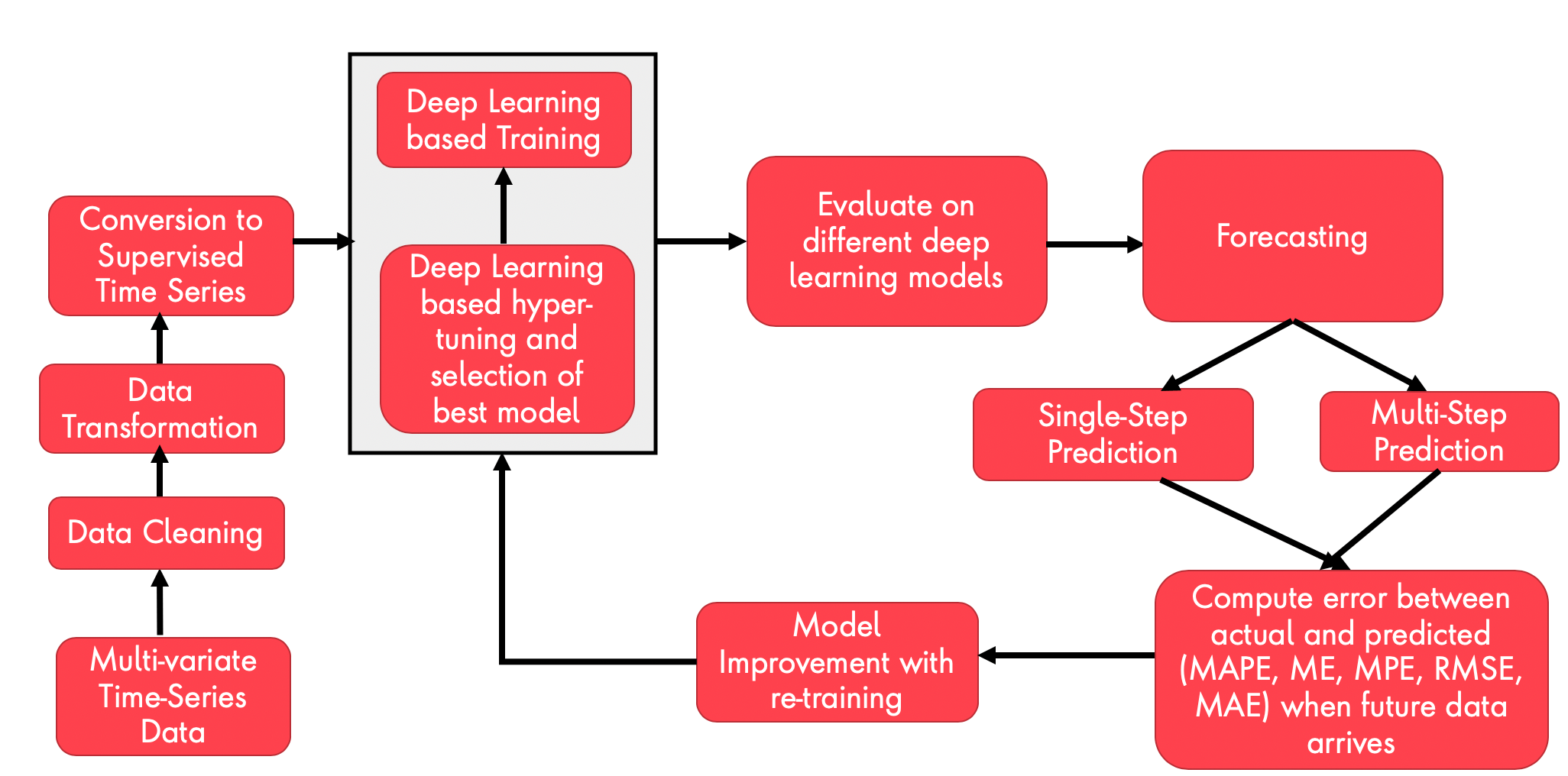• Feeding Multi-variate data from a single source or from aggregated sources available directly from the cloud or other 3rd-party providers into the ML modeling data ingestion system.
• Cleaning, preprocessing, and feature engineering of the data involving scaling and normalization.
• Conversion of the data to a supervised time-series.
• Feeding the data to a deep learning training source that can train different time-series models like LSTM, CNN, BI-LSTM, CNN+LSTM using different combinations of hidden layers, neurons, batch-size, and other hyper-parameters.
• Forecasting based on near term or far distant term in future either using Single-Step or Multi-Step Forecasting respectively
• Evaluation of some of the error metrics like (MAPE, MAE, ME, RMSE, MPE) by comparing it with the actual data, when it comes in
• Re-training the model and continuous improvements when the threshold of error exceeds.

As Delhi had high Covid-19 cases, here we model different DL models for the “DELHI” State (National Capital of India). Further, we keep the scope of dates from 25th March to 6th June 2020. Data till 29th April has been used for Training, whereas from 30th April to 6th June has been used for testing/prediction.

#### Here, we have selected features mostly related to the availability of medical facilities like hospitals, ICU beds, the number of testing facilities, and a number of cured/discharged/migrated/quarantined centers.

stateName = unique_states
dataset =list_state_all
dataset = dataset.sort_values(by='Date', ascending=True)
dataset = dataset[(dataset['Date'] >= '2020-03-25') & (dataset['Date'] <= '2020-06-06')]

daterange = dataset['Date'].values
no_Dates = len(daterange)

dateStart = daterange
dateEnd = daterange[no_Dates - 1]

dataset = dataset[['Total Confirmed cases','Death',
'Cured/Discharged/Migrated', 'coronaenquirycalls',
'cumulativepeopleinquarantine', 'negative', 'numcallsstatehelpline',
'numicubeds', 'numisolationbeds', 'numventilators',
'populationncp2019projection', 'positive',
'testpositivityrate',
'testspermillion', 'testsperpositivecase', 'testsperthousand',
'totalpeoplereleasedfromquarantine', 'totalppe', 'totaltested',
'unconfirmed', 'Active Cases']]

`` ``

As we have 22 features in total, we ensure each of the input features are initially scaled and then are time-shifted by one unit (t+1) th output for t th input to yield in 22 input features plus one output predicted outcome, i.e. The Number of Active Cases. The rest of the columns are dropped. The below code snippet explains that in detail.

## Feature Scaling

This becomes very important given, as in this current problem scope the features vary in the range too much, (10 to 1000000)

#no_features = 22
no_features = np.shape(dataset)-1
print("No of features", no_features)
values = dataset.values

# ensure all data is float
values = values.astype('float32')
print(np.shape(values))
# normalize features
scaler = MinMaxScaler(feature_range=(0, 1))
scaled = scaler.fit_transform(values)

reframed = series_to_supervised(scaled, 1, 1)
# drop columns we don't want to predict
print(np.shape(reframed))

## Convert Time-Series to Supervided Dataset

`This procedure is known as a one-step prediction in time series which uses lagged (one) observations (e.g. t-1) as input variables to forecast the current time step (t). This ensures all series are stationary with differencing and seasonal adjustment.`

# # convert series to supervised learning
def series_to_supervised(data, n_in=1, n_out=1, dropnan=True):
n_vars = 1 if type(data) is list else data.shape
df = pd.DataFrame(data)
cols, names = list(), list()
# input sequence (t-n, ... t-1)
for i in range(n_in, 0, -1):
cols.append(df.shift(i))
names += [('var%d(t-%d)' % (j + 1, i)) for j in range(n_vars)]
# forecast sequence (t, t+1, ... t+n)
for i in range(0, n_out):
cols.append(df.shift(-i))
if i == 0:
names += [('var%d(t)' % (j + 1)) for j in range(n_vars)]
else:
names += [('var%d(t+%d)' % (j + 1, i)) for j in range(n_vars)]
# put it all together
agg = pd.concat(cols, axis=1)
agg.columns = names
# drop rows with NaN values
if dropnan:
agg.dropna(inplace=True)
return agg

After the redundant/un-necessary columns are dropped (24,25,26,27,28,29,30,31,32,33,34,35,36,37,38,39,40,41,42,43,44,45) the entire dataset is split into training and testing dataset in the ratio of 60%:40%, and then we apply different deep learning techniques.

As we train only on the basis of 22 features and predict one output, columns starting from 24 to 45 are dropped.

reframed.drop(reframed.columns[[24,25,26,27,28,29,30,31,32,33,34,35,36,37,38,39,40,41,42,43,44,45]], axis=1, inplace=True)

# split into train and test sets
values = reframed.values
split_factor = int(dataset.shape*0.6)
print(split_factor)
train = values[:split_factor, :]
test = values[split_factor:, :]

print(np.shape(train))
print(np.shape(test))

# split into input and outputs
train_X, train_y = train[:, :-1], train[:, -1]
test_X, test_y = test[:, :-1], test[:, -1]

print(train_X.shape, train_y.shape, test_X.shape, test_y.shape)

# reshape input to be 3D [samples, timesteps, features]
train_X = train_X.reshape((train_X.shape, 1, train_X.shape))
test_X = test_X.reshape((test_X.shape, 1, test_X.shape))
print(train_X.shape, train_y.shape, test_X.shape, test_y.shape)

print(train_X.shape, train_X.shape)

The figure typically depicts a multi-layered stacked LSTM based Neural Network.

## LSTM

The following code snippet demonstrates how we train an LSTM model, plot the training and validation loss, before making a prediction.

`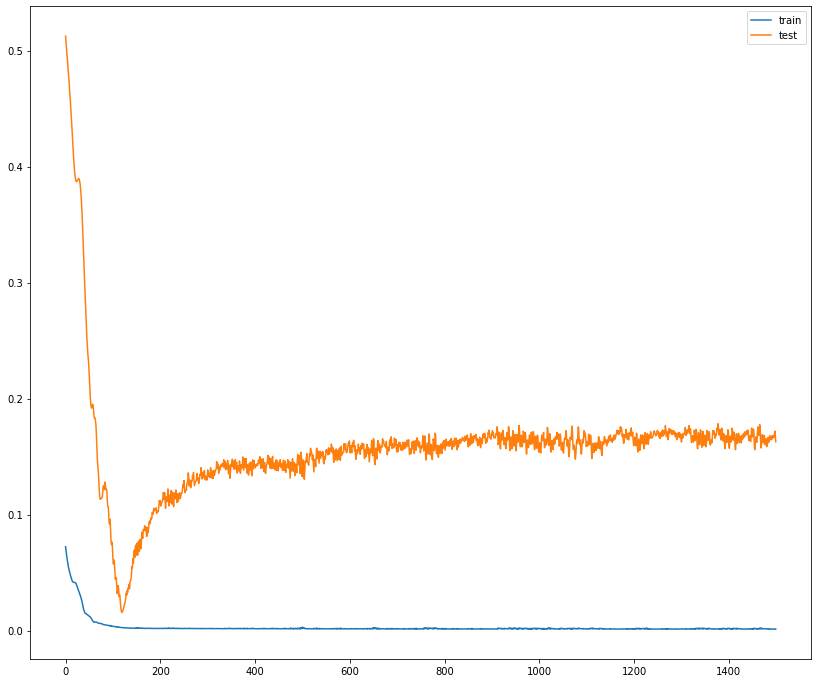Training vs Validation Loss`

This code snippet shows a mechanism to compute the error metrics and inverse scale the predicted outcome.

# design Stacked LSTM networks
model = Sequential()

# fit network
history = model.fit(train_X, train_y, epochs=1500, batch_size=72, validation_data=(test_X, test_y), verbose=2, shuffle=False)
# plot history
plt.figure(figsize=(14,12))
plt.plot(history.history['loss'], label='train')
plt.plot(history.history['val_loss'], label='test')
plt.legend()
plt.show()

# make a prediction
y_predict = model.predict(test_X)
test_X = test_X.reshape((test_X.shape, test_X.shape))
print(train_X.shape, train_y.shape, test_X.shape, test_y.shape)LSTM Model Prediction

## Bi-Directional LSTM

As we know LSTM (Uni-directional) preserves information from inputs to the outputs that have already passed through it using the hidden state.

On the contrary, bidirectional will run inputs in two ways, one from past to future and one from future to past. This kind of LSTM that runs backward to preserve information from the future and using the two hidden states combined, it is able in any point in time to preserve information from both past and futureSource

The following code snippet demonstrates how we train a Bi-LSTM model, plot the training and validation loss, before making a prediction.

train = values[:split_factor, :]
test = values[split_factor:, :]

print(np.shape(train))
print(np.shape(test))

# split into input and outputs
train_X, train_y = train[:, :-1], train[:, -1]
test_X, test_y = test[:, :-1], test[:, -1]

print(train_X.shape, train_y.shape, test_X.shape, test_y.shape)

# reshape input to be 3D [samples, timesteps, features]
train_X = train_X.reshape((train_X.shape, 1, train_X.shape))
test_X = test_X.reshape((test_X.shape, 1, test_X.shape))
print(train_X.shape, train_y.shape, test_X.shape, test_y.shape)

print(train_X.shape, train_X.shape)

# design Stacked LSTM networks/Bi-directional LSTM networks
model = Sequential()

# fit network
history = model.fit(train_X, train_y, epochs=1500, batch_size=72, validation_data=(test_X, test_y), verbose=2, shuffle=False)

`The below figure illustrates the Actual vs Predicted Outcome of Bi-LSTM model , after the predicted outcome has been inverse-transformed (to remove the effect of scaling).`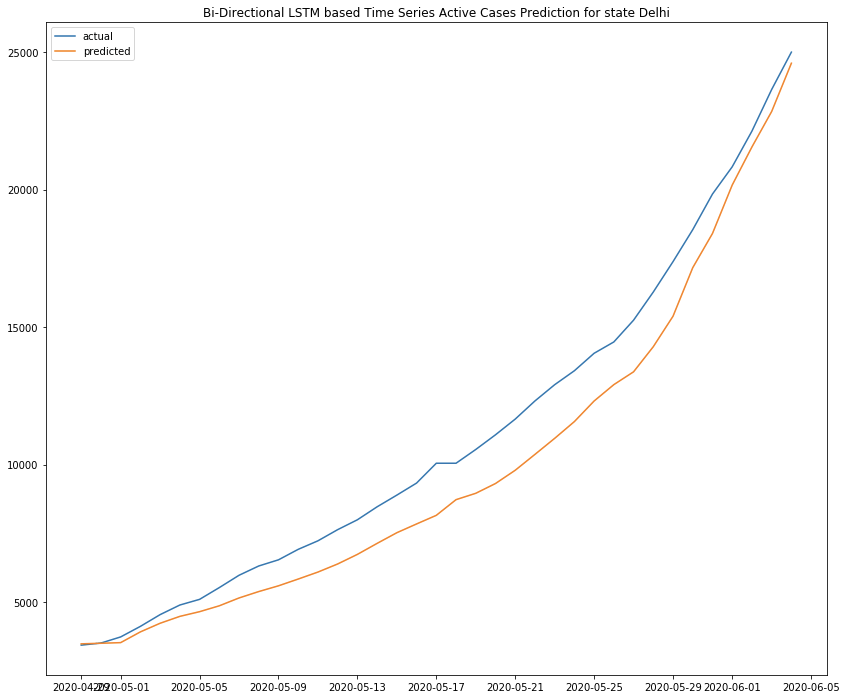BI-LSTM Model Prediction

## CNN (Convolution Neural Network)

We also used CNN for evaluating the model performance for single-step time-series prediction.

The following code snippet demonstrates how we train a CNN model, plot the training and validation loss, before making a prediction.

train_X, train_y = train[:, :-1], train[:, -1]
test_X, test_y = test[:, :-1], test[:, -1]

print(train_X.shape, train_y.shape, test_X.shape, test_y.shape)

# reshape input to be 3D [samples, timesteps, features]
train_X = train_X.reshape((train_X.shape, train_X.shape, 1))
test_X = test_X.reshape((test_X.shape, test_X.shape, 1))
print(train_X.shape, train_y.shape, test_X.shape, test_y.shape)

#CNN
model = Sequential()
model.summary()

#fit model
history =model.fit(train_X, train_y, epochs=1500, batch_size=72, validation_data=(test_X, test_y), verbose=2,shuffle=False)

## Trained Model for Prediction

# make a prediction
y_predict = model.predict(test_X)
test_X = test_X.reshape((test_X.shape, test_X.shape))
print(train_X.shape, train_y.shape, test_X.shape, test_y.shape)

` `

## Inverse Transofrm Predictions and Computation of Error

inv_y_predict = concatenate((y_predict, test_X[:, -(no_features):]), axis=1)

inv_y_predict = scaler.inverse_transform(inv_y_predict)
inv_y_predict = inv_y_predict[:, 0]
# invert scaling for actual
test_y = test_y.reshape((len(test_y), 1))
inv_y = concatenate((test_y, test_X[:, 1:]), axis=1)
inv_y = scaler.inverse_transform(inv_y)
inv_y = inv_y[:, 0]
# calculate RMSE
rmse = np.sqrt(mean_squared_error(inv_y, inv_y_predict))
print('Test RMSE: %.3f' % rmse)

pred_len = len(inv_y_predict)
print(pred_len)
dateEnd = daterange[split_factor+1]
print(dateEnd)
pred_index= pd.date_range(start=dateEnd, periods=pred_len, freq='D')
#print(pred_index)

inv_y_actual = pd.Series(inv_y, pred_index)
inv_y_predicted = pd.Series(inv_y_predict, pred_index)

` `
`The below figure illustrates the Actual vs Predicted Outcome of CNN model after the predicted outcome has been inverse -transformed (to remove the effect of scaling).`CNN Model Prediction

## CNN + LSTM

Here we have used Conv1d with TimeDistributed Layer, which is then fed to a single layer of LSTM, to predicted different sequences, as illustrated by the figure below.

The CNN model is built first, where each layer in the CNN model is wrapped in a TimeDistributed layer, and then added to the LSTM model.

However, the other alternative approach could be used to construct the CNN model first, then add it to the LSTM model by wrapping the entire sequence of CNN layers in a TimeDistributed layer.

TimeDistributed Layer is primarily used to present several sets of data (say sequences/mages) that are chronologically ordered to detect trends/ movements, actions, directions.# split into input and outputs
train_X, train_y = train[:, :-1], train[:, -1]
test_X, test_y = test[:, :-1], test[:, -1]
print(train_X.shape, train_y.shape, test_X.shape, test_y.shape)

#LSTM + CNN
subsequences = 1
timesteps = train_X.shape
X_train_series_sub = train_X.reshape((train_X.shape, subsequences, timesteps, 1))
X_valid_series_sub = test_X.reshape((test_X.shape, subsequences, timesteps, 1))
print('Train set shape', X_train_series_sub.shape)
print('Validation set shape', X_valid_series_sub.shape)

model = Sequential()
model.add(TimeDistributed(Conv1D(filters=64, kernel_size=1, activation='relu'), input_shape=(None, X_train_series_sub.shape, X_valid_series_sub.shape)))

history = model.fit(X_train_series_sub, train_y, validation_data=(X_valid_series_sub, test_y), epochs=1500, verbose=2)

The prediction and inverse scaling help to yield the actual predicted outcomes.

#Prediction (LSTM + CNN)
yhat = model.predict(X_valid_series_sub)
print(yhat)
test_X = X_valid_series_sub.reshape((X_valid_series_sub.shape, X_valid_series_sub.shape))

inv_y_predict = concatenate((y_predict, test_X[:, -(no_features):]), axis=1)

inv_y_predict = scaler.inverse_transform(inv_y_predict)
inv_y_predict = inv_y_predict[:, 0]

# invert scaling for actual
test_y = test_y.reshape((len(test_y), 1))
inv_y = concatenate((test_y, test_X[:, 1:]), axis=1)
inv_y = scaler.inverse_transform(inv_y)
inv_y = inv_y[:, 0]

# calculate RMSE
rmse = np.sqrt(mean_squared_error(inv_y, inv_y_predict))
print('Test RMSE: %.3f' % rmse)

pred_len = len(inv_y_predict)
print(pred_len)
dateEnd = daterange[split_factor+1]
print(dateEnd)
pred_index= pd.date_range(start=dateEnd, periods=pred_len, freq='D')
#print(pred_index)

inv_y_actual = pd.Series(inv_y, pred_index)
inv_y_predicted = pd.Series(inv_y_predict, pred_index)

`The below figure illustrates the Actual vs Predicted Outcome of stacked LSTM and CNN model after the predicted outcome has been inverse -transformed (to remove the effect of scaling).`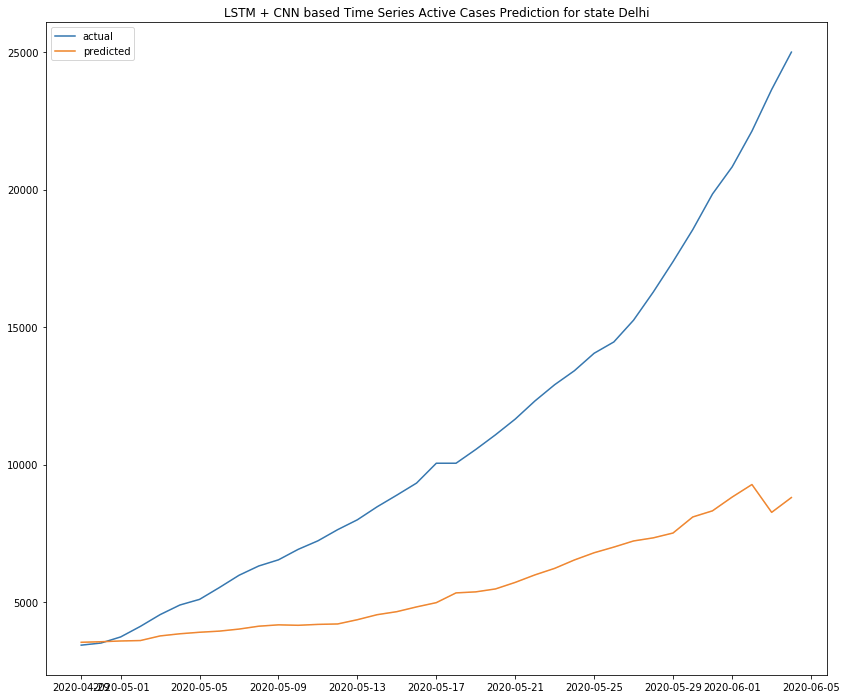LSTM with CNN

## Training and Validation Loss

Epoch 1494/1500
58/58 - 0s - loss: 3.2615e-06 - val_loss: 0.0056
Epoch 1495/1500
58/58 - 0s - loss: 3.3479e-06 - val_loss: 0.0056
Epoch 1496/1500
58/58 - 0s - loss: 3.3705e-06 - val_loss: 0.0053
Epoch 1497/1500
58/58 - 0s - loss: 3.2291e-06 - val_loss: 0.0054
Epoch 1498/1500
58/58 - 0s - loss: 3.0793e-06 - val_loss: 0.0056
Epoch 1499/1500
58/58 - 0s - loss: 3.8484e-06 - val_loss: 0.0055
Epoch 1500/1500
58/58 - 0s - loss: 3.8213e-06 - val_loss: 0.0054

```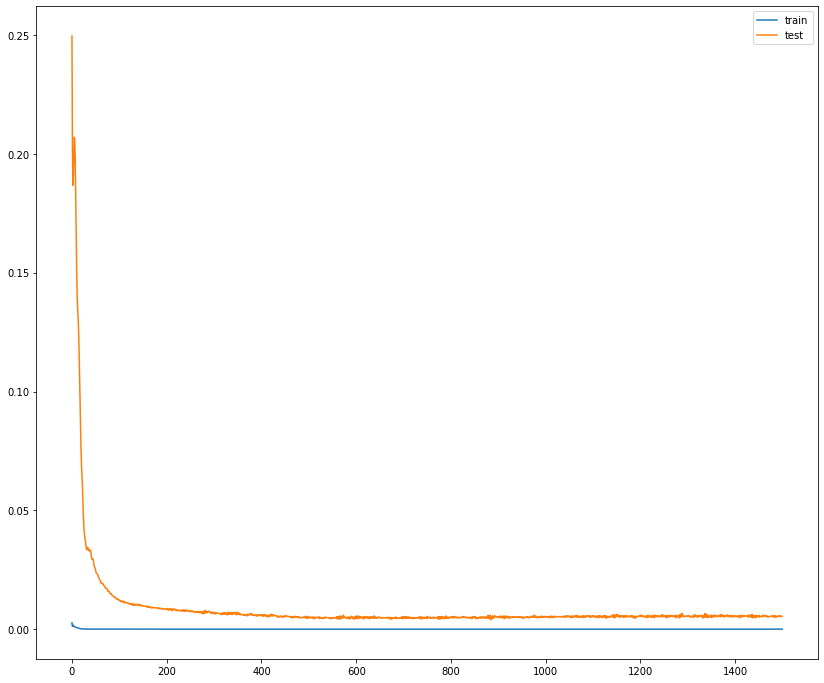Train vs Validation Loss

```

The following table depicts the computed RMSE metrics for each of the deep learning models.

 Deep Learning Method RMSE LSTM 5262.208 BI-LSTM 804.197 Stacked LSTM 2730.476 CNN 8634.9 LSTM + CNN 8634.9

Error Metrics of Deep Learning-based Models

## Conclusion

Here we see bi-directional LSTM works the best, followed by multiple stacked layers of LSTM and single LSTM layer. This is just a basic study and results might differ based on the dataset. In the next blog (series 2 ) we will see different multi-step prediction results.

More extensive hyper-parameter tuning is needed along with dynamic data featuring a change in medical facilities and supplies.

For complete source code check out https://github.com/sharmi1206/covid-19-analysis

## Acknowledgments

Special thanks to machinelearningmastery.com. as some of the concepts have been taken from there.

## References

Views: 1811

Tags: dsc_biotech, dsc_ml, dsc_tagged

Comment